Document Type: Original Research Article

Authors

1 Chemistry Department, Faculty of Science, Mansoura University, 35516-Mansoura, Egypt

2 Chemistry Department, Faculty of Science, Damietta University, Damietta, Egypt

10.29088/SAMI/PCBR.2018.1.1928

Abstract

The molar solubility for 2-amino-4,5-dimethylthiophene-3-carboxamide  (ADTC) in pure ethanol and mixed ethanol (EtOH)- water solvents were measured at five different temperatures, 293.15, 298.15, 303.15, 308.15, and 313.15 K in the used mixed solvents, the solubility were increase by increase in the mole fraction of ethanol in the mixtures and increase of temperature due to more salvation parameters. All the solvation and thermodynamic parameters for ADTC in mixed EtOH+ H2O solvents were evaluated like solubility products, Gibbs free energies, enthalpies and entropies of solvation and discussed. Theoretical calculations for (ADTC) were done quantum mechanically by using Gaussian on set of calculations in ethanol for evaluating the different thermodynamic parameters.

Graphical Abstract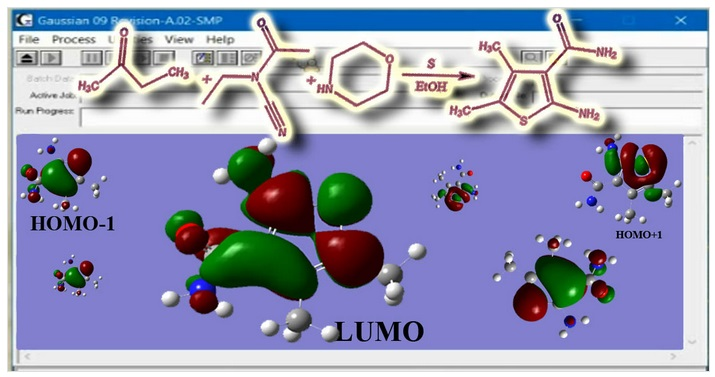Highlights

• Experimetal evaluation of the thermodynamic parameters from solubility measurements.
• Theoretical calculation of the thermodynamic parameters of the used material.
• Trying to explain the experimental values theoretically.

Keywords

###### ##### Full Text

1. Introduction

Co-solvents (any solvent other than the first solvent) are known to affect the solubility of solutes in solutions. Theory of effects of co-solvents is desirable in order to understand  the  molecular  interactions  for  changes  in solubility and improve the properties of solutions . The Kirhwood – Buff theory (KBT) of solutions  has been used to explain the effects of co-solvents on the solubility of solutes in terms of intermolecular distributions [2, 3]. KBT is an exact theory of solution mixtures and provide information for solute understanding effects . The general idea for solubility of molecules to explain the local microscopic experimental results. Also studying the intermediates for salvation processes are also needed. Also explaining the chemical potentials of the solute can also be expressed by statistical thermodynamic calculations [3, 4]. Studies for a quantitative description of metal ion–solvent binding are of immense importance in various fields of chemistry, physics and biology, as well as in the technological development of various practical separation processes. The subject has drawn across the board consideration [5-14].

Experimental

2.1.   Materials

The chemicals used 2-butanone, ethyl cyanoacetamide; sulfur and morpholine are provided from Sigma Aldrich chemical company. All chemicals are used without purification to reserve them in their states. Absolute ethanol provided from El-Nasr Chemical and Pharmaceutical Company was used. High conductivity water was used.

2.2.   Synthesis

The starting 2-amino-4,5-dimethylthiophene-3-carboxamide(ADTC) 4 was obtained via Gewald reaction by condensation of 2-butanone 1 with ethyl cyanoacetamide 2 in the presence of elemental sulphur and morpholine 3 (base) as in Scheme 1. The structures were confirmed by various spectroscopic techniques, including IR, 1H NMR and mass spectroscopy. The IR spectra of compound showed characteristic absorption bands in the region within υ = 3411-3163 cm–1 due to the stretching vibrations of 2 NH2 groups. The bands in the region within υ = 1670 cm–1 are due to the stretching vibrations of carbonyl group. The absorption bands in the region within υ = 1265-1280 cm–1 are assigned to the stretching vibrations of 2 CH3 groups.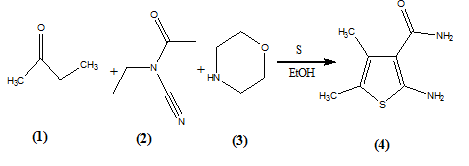Scheme 1. 2-amino-4,5-dimethylthiophene-3-carboxamide (4) was obtained via Gewald reaction by condensation of 2-butanone (1) with ethyl cyanoacetamide (2) in the presence of elemental sulfur and morpholine (3).

Beside the expected signals in the 1H NMR spectrum of compound, it displayed a characteristic broad signal at d 12.97 and 13.15 ppm assigned to 2NH2 groups and  a broad signal d 2.30 due to the methyl group protons. The mass spectrum of the compound showed the molecular ion peaks at m/z 172 (M++2), 171 (M++1), 170 (M+) which is in agreement with molecular formula of the compound C7H10N2OS.

2.3.     Preparation of saturated solutions.

The saturated solutions of 2-amino-4,5-dimethylthiophene-3-carboxamide (ADTC) in mixed EtOH-H2O solvents were prepared by dissolving solid material (ADTC) in closed test tubes containing different mole fractions of ethanol-water solvents. The tubes were put in water thermostat of type assistant for a period of one day at temperatures 293.15, 298.15, 303.15, 308.15, and 313.15 K till equilibrium reached. The solubility of (ADTC) was measured by taking 1ml of each saturated solution putting in a small weighed beaker (10ml) and evaporate under I.R. Lamp till dryness and then weights [15-17].

3. Results and discussion

3.1.Calculation of thermodynamic parameters of solvation

3.1.1.Molal solubility

The molal solubility of compound ADTC in mixed ethanol – water solvent was evaporated till dryness in small beaker.

Molal solubility (S) = (W×1000)/(M.wt)(d)                    (1)

W is the weight of the residue in the beaker. M.wt is the molecular weight of the compound. d is the density of solvent.

3.1.2. Solubility product

The solubility product of compound (ADTC) was calculated by using   (eq. 2)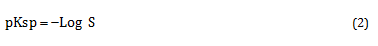Where Pksp is the solubility product and S is the molal solubility and all the data at different temperatures were calculated in tables 1-5.

Table 1: Molal solubility (S), Log S, log activity coefficient (log γ), solubility product (pKsp), Gibbs free energy (ΔG) , transfer Gibbs free energy (ΔGt) for ADTC at different mole fraction (Xs) and different concentrations of EtOH in EtOH – H2O mixtures at 293.15 K.

 EtOH vol. % Xs S Log S log pKsp ΔGs in (kJ mol-1) ΔGt in (kJ mol-1) 0 0 0.0163 -1.788 -0.0507 3.6782 20.646 0.000 20 0.0717 0.0263 -1.579 -0.06299 3.2846 18.436 -2.209 40 0.1708 0.0371 -1.43 -0.07493 3.0103 16.897 -3.749 60 0.3166 0.0707 -1.151 -0.10013 2.502 14.043 -6.602 80 0.5527 0.6334 -0.198 -0.21273 0.8221 4.6144 -16.031 100 1 1.9717 0.2948 -0.63966 0.6897 3.871 -16.775

Table 2: Solubility S, Log Solubility, log activity coefficient (log γ), solubility product (pKsp), Gibbs free energy (ΔG) , transfer Gibbs free energy (ΔGt) for ADTC at different mole fraction (Xs) and different concentrations of EtOH in EtOH – H2O mixtures at 298.15 K.

 EtOH vol. % Xs S Log S log pKsp ΔGs in (kJ mol-1) ΔGt in   (kJ mol-1) 0 0 0.0189 -1.724 -0.05397 3.5566 20.304 0.000 20 0.0717 0.032 -1.495 -0.06815 3.1265 17.848 -2.456 40 0.1708 0.0425 -1.372 -0.07903 2.9014 16.563 -3.740 60 0.3166 0.11 -0.959 -0.11511 2.1475 12.259 -8.044 80 0.5527 0.6974 -0.157 -0.21781 0.7487 4.2739 -16.030 100 1 2.0133 0.3039 -0.65124 0.6947 3.9657 -16.338

Table (3): Solubility S, Log Solubility, log activity coefficient (log γ), solubility product (pKsp), Gibbs free energy (ΔG) , transfer Gibbs free energy (ΔGt) for ADTC at different mole fraction (Xs) and different concentrations of EtOH in EtOH – H2O mixtures at 303.15 K.

 EtOH vol. % Xs S Log S log pKsp ΔGs in (kJ mol-1) ΔGt in   (kJ mol-1) 0 0 0.0195 -1.709 -0.05534 3.5291 20.484 0.000 20 0.0717 0.0386 -1.413 -0.07351 2.9728 17.256 -3.229 40 0.1708 0.045 -1.347 -0.08084 2.856 16.577 -3.907 60 0.3166 0.1141 -0.943 -0.1176 2.121 12.311 -8.173 80 0.5527 0.7717 -0.113 -0.22356 0.6723 3.9022 -16.582 100 1 2.2286 0.348 -0.66681 0.6376 3.7007 -16.784

Table 4: Solubility S, Log Solubility, log activity coefficient (log γ), solubility product (pKsp), Gibbs free energy (ΔG) , transfer Gibbs free energy (ΔGt) for ADTC at different mole fraction (Xs) and different concentrations of EtOH in EtOH – H2O mixtures at 308.15 K

 EtOH vol. % Xs S Log S log pKsp ΔGs in (kJ mol-1) ΔGt in   (kJ mol-1) 0 0 0.0215 -1.669 -0.0581177 3.4533 20.375 0.000 20 0.0717 0.0389 -1.41 -0.07705477 2.9747 17.551 -2.824 40 0.1708 0.0467 -1.331 -0.08757112 2.8373 16.74 -3.635 60 0.3166 0.119 -0.925 -0.13776618 2.1246 12.536 -7.839 80 0.5527 0.906 -0.043 -0.28244954 0.6506 3.8388 -16.536 100 1 2.4116 0.3823 -0.6889448 0.6133 3.6186 -16.756

Table 5: Solubility S, Log Solubility, log activity coefficient (log γ), solubility product (pKsp), Gibbs free energy (ΔG) , transfer Gibbs free energy (ΔGt) for ADTC at different mole fraction (Xs) and different concentrations of EtOH in EtOH – H2O mixtures at 313.15 K

 EtOH vol. % Xs S Log S log pKsp ΔGs in (kJ mol-1) ΔGt in   (kJ mol-1) 0 0 0.0234 -1.631 -0.0607354 3.3829 20.284 0.000 20 0.0717 0.043 -1.367 -0.0818743 2.8971 17.371 -2.913 40 0.1708 0.0499 -1.302 -0.093041 2.7904 16.731 -3.552 60 0.3166 0.1236 -0.908 -0.1519647 2.1198 12.71 -7.574 80 0.5527 0.933 -0.03 -0.2996969 0.6597 3.9553 -16.328 100 1 2.6349 0.4208 -0.727544 0.6136 3.6788 -16.605

Table 6: Enthalpy change (ΔH ) and entropy change (ΔS ) of solvation  for ADTC in (EtOH–H2O)  mixed solvents at different temperatures in (kJ mol-1).

 EtOH vol. % Xs Δ H (kJ mol-1) Δ S 293.15K Δ S 298.15K Δ S 303.15K Δ S 308.15K Δ S 313.15K 0 0 24.423 0.0129 0.0138 0.0130 0.0131 0.0132 20 0.0717 32.757 0.0489 0.0500 0.0511 0.0493 0.0491 40 0.1708 17.797 0.0031 0.0041 0.0040 0.0034 0.0034 60 0.3166 28.094 0.0479 0.0531 0.0521 0.0505 0.0491 80 0.5527 14.991 0.0354 0.0359 0.0366 0.0362 0.0352 100 1 8.222 0.0148 0.0143 0.0149 0.0149 0.0145

3.1.3.    Activity coefficient

The activity coefficients were calculated using Debye – Hückel equation (3)  and their values and the solubilities are given in tables 1 &2.

log  = - 0.5062 x (S)0.5                                                           (3)

3.1.4.    Free energy of solvation

From the solubility products the Gibbs free energies of solvation and the transfer Gibbs free energies from water to mixed solvents were calculated by using equations 4 & 5.

Δ Gs = RT ln pKsp                                                                                          (4)

Δ Gt = Δ GS – Δ Gw                                                                                         (5)

All the data are tabulated in tables 1-5 at different temperatures and shown in figures 3 &4 .

3.1.5.              Enthalpies and entropies of solvation

Enthalpies can be calculated by drawing a relation between log Ksp for ADTC against 1/T, the slope=-∆H/2.303R. So, the entropy (ΔS) of solvation for ADTC concentration solutions can be calculated using Gibbs-Helmholtz equation [19-25].

Δ G = Δ H - T Δ S                                                                                        (6)

All the data are tabulated in table 6. The solvation experimental thermodynamic parameters ΔG and ΔS for ADTC in mixed EtOH-H2O solvents are increased with increase in the percentage (mole fraction) of ethanol in the mixed solvents favoring more solute-solvent interactions.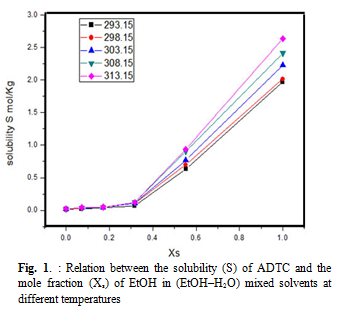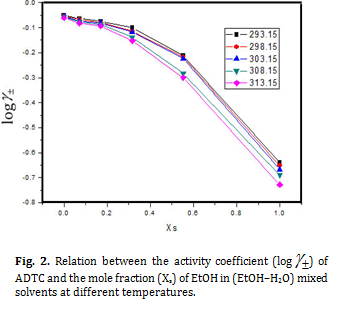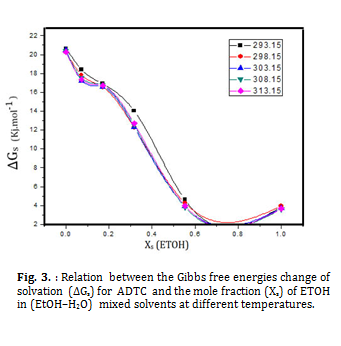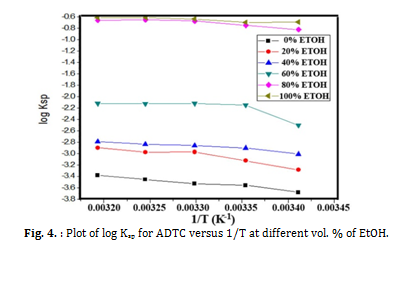The enthalpy changes are decrease with increase of the ethanol percentage favoring more endothermic solvation process. Little effect of temperature is noticed experimentally for solvation of ADCT in mixed EtOH-H2O solvents due to small entropies of solvation and the endothermic reaction happened.

3.2.     Theoretical Thermochemistry of the new Schiff base, ADTC

The equation used entropy, energy and heat capacity resulting from the calculation frequencies using Gaussian 09 package are given below [26-30]: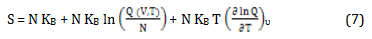Where N = n NA , KB = R , NA is Avogadro's number , R is gas constant and Q is the partition function changing into logarithm we obtain: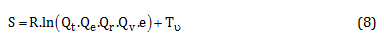Where Q is the partition function (total), the Qt, Qe, Qr, Qv, denote, the translational, electronic, rotational and vibrational partition function. The thermal energy can be obtained from the partition function: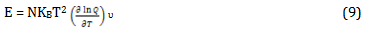The heat capacity can be obtained by using equation 10 :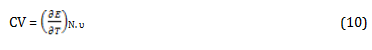Where CV is the heat capacity at constant volume,  is the difference in internal energy ,  is the difference in temperatures used, N is the number of particles, . υ is the vibrational quantum number The above equations will be used for estimation the available thermodynamic for the organic compounds used from the evaluated partition functions. The calculations were done from the contributions for the translation, rotational motion and electronic contributions from vibrational mode of contribution.The data were obtained from frequency analysis by the need or partition function. For vibrational motion, choosing the first vibrational energy K, level to be zero level, the corresponding partition function is expressed as [31, 32].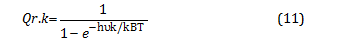Where KB is Baltzmann constant (KB =1.38066 X 10-23 J/K), h is planck's constant (h=6.626 x 10-23 J.S) and hυk / KBT = v, k is defined as vibrational frequency of mode k. The zero of energy is defined as the fully dissociated limit (free electrons and bore nuclei), at absolute zero temperature, which is the small motion of molecules and is called “the zero-point vibrational energy” (ZPVE or ZPE). The ZPVE must be added to obtain energy at absolute zero temperature (T=0 K). For all the 3N-6 (3N-5 for linear molecules) vibrational modes, the total ZPVE [28-30]is: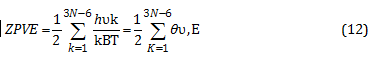The ZPVE is calculated for compounds using the data are given in table (7, 8 and 9) for the different solutions [33, 34].

3.2.1.     Different contributions of Motions

The different types of motions were theoretically studied for the schiff bases and summarized in the next text.

3.2.1.1.                   Contributions from translation

The translational partition function is used to calculate the translational entropy (which donate the factor e which comes from Stirling's approximation [30-36]: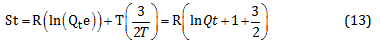The contribution to internal energy is: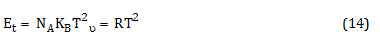Finally, the heat capacity at constant volume is given by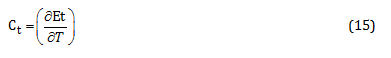3.2.1.2.                   Contributions from electronic motion

The contributions can be calculated from electronic partition function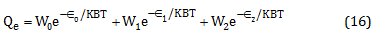Where w is the degeneracy of energy level, En is the energy in n-th level.

The entropy due to electronic motion is: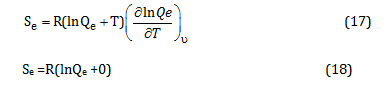Since there are no temperature dependent terms in partition functions, the electronic capacity and internal energy due to electronic motion are both zero.

3.2.1.3.                   Contribution from rotational motion:

For linear molecules, the rotational partition function is: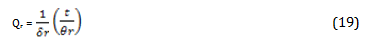Where r = h2 / 8 2 I kB , I is the moment of inertia. r is the rotational diameter. The rotational contribution to the entropy is: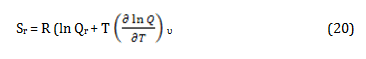The contribution to rotation for for internal energy is: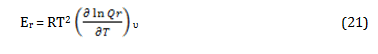And the contribution to the heat capacity is: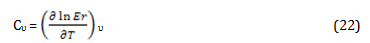For our general case which is nonlinear, the rotational energy and heat capacity are: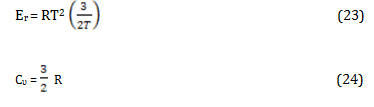3.2.1.4.                    Contributions from vibrational motion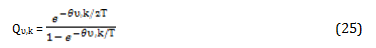The total entropy, energy and Cυ from  the vibrational partition function are: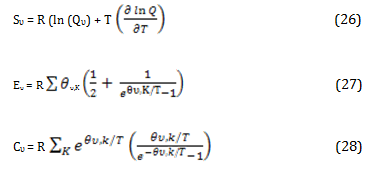3.2.1. Thermochemistry studies of 2-amino-4,5-dimethylthiophene-3-carboxamide (ADTC)

Different partition functions (Q) values for compound ADTC in ethanol were studied by three different methods i.e. Hartree-fock 6-311g(d,p), PM3 and AM1 and tabulated in table 7. As concluded, the vibration partition function (Q) values for all ADTC are greater than transitional and rotational values. In table 8, Different quantum chemical results for compound ADTC in ethanol were calculated by three different methods (Hartree-Fock 6-311g(d,p), PM3 and AM1). The dipole moment, HOMO and LUMO energy levels are also calculated for ADTC in the table. As shown in the table 9, the Sum of electronic and zero point energies, Sum of electronic and thermal energies, Sum of electronic and thermal enthalpies and Sum of electronic and thermal free energies for ADTC in ethanol by three different methods were tabulated. The basis set used for calculations is 6-311g for Hartree-Fock method. Electronic, translational and rotational partition functions are calculated for ADTC in pure ethanol solvent. Most of  the data obtained theoretically are approximately similar other than parameters like the total energy of ADTC, HOMO, LUMO energies.

Table. 7.Different partition functions (Q) values for compound ADTC in ethanol by using three different methods (Hartree-fock 6-311g(d,p), PM3 and AM1).

 Hartree-fock 6-311g PM3 AM1 Q Ln (Q) Q Ln (Q) Q Ln (Q) TotalBot 0.307740D-64 -148.543946 0.213198D-57 -132.792885 0.358527D-60 -139.180856 Total V=0 0.121789D+18 39.341070 0.238734D+19 42.316710 0.185038D+18 39.759339 Vib (Bot) 0.331913D-78 -180.704520 0.225949D-71 -164.970988 0.390508D-74 -171.331603 Electronic 0.100000D+01 0.000000 0.100000D+01 0.000000 0.100000D+01 0.000000 Translational 0.871617D+08 18.283275 0.871617D+08 18.283275 0.871617D+08 18.283275 Rotational 0.106374D+07 13.877299 0.108255D+07 13.894828 0.105334D+07 13.867472

Table. 8. Different quantum chemical results for compound ADTC in ethanol by three different methods (Hartree-fock 6-311g(d,p), PM3 and AM1).

 Hartree-fock 6-311g PM3 AM1 Eps 24.852000 20.493000 24.852000 SCF Done (A.U.) E(RHF)= -852.330476048 E(RPM3) = -0.479586987603E-01 E(RAM1) = -0.604958234463E-01 Dipole moment (Debye) 4.6889 2.9204 3.4629 HOMO -0.29205 -0.32233 -0.31447 LUMO 0.12374 -0.01781 - 0.00573 KE 8.492668572995D+02 -3.860527198823D+01 -3.795418230410D+01 Rotational temperatures 0.06745 K 0.06596 K 0.06767 K Rotational constants (GHZ) 1.40541 1.37434 1.40991 Exact polarizability 159.455 144.205 163.291 Zero-point vibrational energy 111.31918 103.74992 106.01950

Stoichiometry   C7H10N2OS, Deg. of freedom    57, Full point group   C1

Table. 9. Theoretical thermodynamic parameters for compound ADTC in ethanol by three different methods (Hartree-fock 6-311g(d,p), PM3 and AM1)

 Hartree-fock 6-311g PM3 AM1 Sum of electronic and zero-point Energies -852.153078 0.117377 0.108457 Sum of electronic and thermal Energies -852.141726 0.130063 0.120356 Sum of electronic and thermal Enthalpies -852.140782 0.131007 0.121300 Sum of electronic and thermal Free Energies -852.190223 0.077422 0.070917

Table 10. Different thermodynamic parameters such as thermal, heat capacity at constant volume CV, entropies for the electronic, translational, rotational and vibrational movement for ADTC in ethanol by applying three different methods

 Hartree-fock 6-311g PM3 AM1 E (Thermal) KCal/mol CV Cal/mol-Kelvin S Cal/mol-Kelvin E (Thermal) KCal/mol CV Cal/mol-Kelvin S Cal/mol-Kelvin E (Thermal) KCal/mol CV Cal/mol-Kelvin S Cal/mol-Kelvin Total 118.442 42.304 104.058 111.710 44.762 112.778 113.486 43.623 106.041 Electronic 0.000 0.000 0.000 0.000 0.000 0.000 0.000 0.000 0.000 Translational 0.889 2.981 41.301 0.889 2.981 41.301 0.889 2.981 41.301 Rotational 0.889 2.981 30.558 0.889 2.981 30.593 0.889 2.981 30.538 Vibrational 116.665 36.343 32.199 109.933 38.801 40.885 111.709 37.661 34.202

Kinetic energy, rotational constant, polarizability, zero point vibrational energy are calculated by applying the three methods and found that they are greater on using Hartree-fock method than others. Sum of electronic+ zero point energies, sum of electronic + thermal energies and sum of electronic+ thermal enthalpies was calculated by using the above mentioned methods and found to be greater using Hartree-fock method than others. The different thermodynamic parameters such as thermal, heat capacity at constant volume CV, entropies for the electronic, translational, rotational and vibrational movement for ADTC in ethanol were also calculated by applying the three different methods theoretical and found to give the greatest values on using PM3 method supporting the activity of ADTC in ethanol medium (table 10).  The electronic gap energy E(LUMO)–E(HOMO)  gave bigger result 0.4157 eV (electron Volt) in  case of Hartree-fock method than other methods indicating the applicability of this compound ADTC as semiconductor.  As shown in Fig. 5, the shape of HOMO and HOMO-1 indicate that HOMO orbitals are full occupied than that of HOMO-1. Also LUMO orbitals are clear to have more orbital free to be occupied than that of LUMO+1orbitals.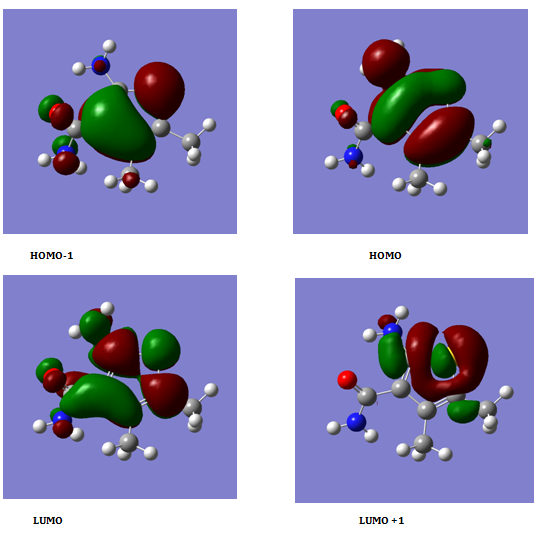Fig. 6. The HOMO-1, HOMO, LUMO, LUMO+1 for ADTC in ethanol.

Conclusions

The solvation experimental thermodynamic parameters ΔG and ΔS for ADTC in mixed EtOH-H2O solvents are increased with increase in the percentage (mole fraction) of ethanol in the mixed solvents favoring more solute-solvent interactions. The enthalpy changes are decreased with increase of the ethanol percentage favoring more endothermic solvation process. Different methods of calculation were proceeded like, Hartree-Fock 6-311g (d,p), PM3 and AM1 fore estimating the different thermodynamic parameters in ethanol. The different thermodynamic parameters like thermal, heat capacity at constant volume CV, entropies for the electronic, translational, rotational and vibrational movement and other theoretical parameters for ADTC in ethanol were also calculated by applying the three different methods theoretical and found to give the grate values supporting the activity of ADTC in ethanol medium. This indicates that the solubility of ADCT is increased by increase in ethanol mole fraction in the mixed solvents.

###### ##### References

1. References

 P.E. Smith and R.M. Mazo. The Journal of Physical Chemistry B, 2008, 112,  7875-7884.

 L.L. Lee. Fluid Phase Equilibria, 1997, 131,  67-82.

 E. Ruckenstein and I. Shulgin. Industrial & engineering chemistry research, 2002, 41,  4674-4680.

 R.M. Mazo. The Journal of Physical Chemistry B, 2006, 110,  24077-24082.

 K. Michaelian and M. Moskovits. Nature, 1978, 273,  135.

 R. Impey, P. Madden and I. McDonald. The Journal of Physical Chemistry, 1983, 87,  5071-5083.

 L.X. Dang, J.E. Rice, J. Caldwell and P.A. Kollman. Journal of the American Chemical Society, 1991, 113,  2481-2486.

 L.X. Dang. The Journal of chemical physics, 1992, 96,  6970-6977.

 H. Ohtaki and T. Radnai. Chemical Reviews, 1993, 93,  1157-1204.

 R. Barnett and U. Landman. Physical review letters, 1993, 70,  1775.

 D. Feller, E.D. Glendening, R.A. Kendall and K.A. Peterson. The Journal of chemical physics, 1994, 100,  4981-4997.

 D.A. Doyle, J.M. Cabral, R.A. Pfuetzner, A. Kuo, J.M. Gulbis, S.L. Cohen, B.T. Chait and R. MacKinnon. science, 1998, 280,  69-77.

 K. Hashimoto and T. Kamimoto. Journal of the American Chemical Society, 1998, 120,  3560-3570.

 A. Pathak, T. Mukherjee and D. Maity. The Journal of chemical physics, 2006, 124,  024322.

 E.A. Gomaa. Thermochimica acta, 1989, 156,  91-99.

 E.M. Abou Elleef and E.A. Gomaa. International journal and innorative technology,, 2013,  308-313

 E.A. Gomaa. American journal of systems of science, 2014, 3,  13-17.

 A.A. El Khouly, E.A. Gomaa and S.M. Abou Elleef. Bulletin of Electrochemistry, 2003, 19,  153-164.

 S.L. Oswal, J.S. Desai, S.P. Ijardar and D.M. Jain. Journal of Molecular Liquids, 2009, 144,  108-114.

 D. Bobicz, W. Grzybkowski and A. Lewandowski. Journal of molecular liquids, 2003, 105,  93-104.

 Y. Marcus, The Properties of Solvents. 1998, London: Wiley.

 E.A. Gomaa, A.H. El-Askalany and M.N.H. Moussa. Asian Journal of Chemistry, 1992, 4,  553-559.

 O. Popovych, A. Gibofsky and D.H. Berne. Analytical chemistry, 1972, 44,  811-817.

 I.M.S. Lampreia and L.A.V. Ferreira. Journal of the Chemical Society, Faraday Transactions, 1996, 92,  1487-1491.

 P.W. Atkins, Physical chemistry. 1978: Oxford University Press

 A.K. Chandra and T. Uchimaru. The Journal of Physical Chemistry A, 2001, 105,  3578-3582.

 P. Kaatz, E.A. Donley and D.P. Shelton. The Journal of chemical physics, 1998, 108,  849-856.

 S.P. Karna, P.N. Prasad and M. Dupuis. The Journal of chemical physics, 1991, 94,  1171-1181.

 L.T. Cheng, W. Tam, S.H. Stevenson, G.R. Meredith, G. Rikken and S.R. Marder. The Journal of Physical Chemistry, 1991, 95,  10631-10643.

 B.P.L. POLAVARAPU. Molecular Physics, 1997, 91,  551-554.

 M. Bendikov, Y. Apeloig, S. Bukalov, I. Garbuzova and L. Leites. The Journal of Physical Chemistry A, 2002, 106,  4880-4885.

 R.G. Parr and W. Yang, Density – Functional Theory of Atoms and Molecules. 1989, New York: Oxford University Press.

 N. Tokitoh, K. Wakita, R. Okazaki, S. Nagase, P. von Ragué Schleyer and H. Jiao. Journal of the American Chemical Society, 1997, 119,  6951-6952.

 E.B. Wilson, J.C. Decius and P.C. Cross, Molecular vibrations: the theory of infrared and Raman vibrational spectra. 1980: Courier Corporation.

 K. Hassler. Spectrochimica Acta Part A: Molecular Spectroscopy, 1981, 37,  541-548.

 K. Wakita, N. Tokitoh, R. Okazaki and S. Nagase. Angewandte Chemie International Edition, 2000, 39,  634-636.